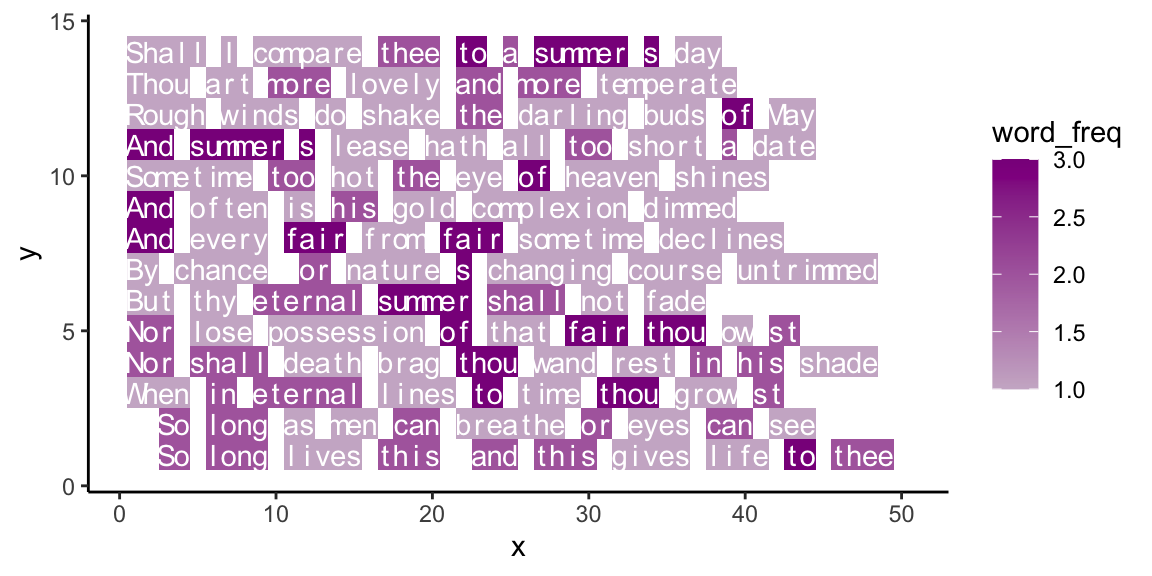## 19.5 ExercisesExercises on R pour l’art:

### 19.5.1 Visualizing word frequency

• Our example of plotting text in Section 19.3 used color to visualize the frequency of each character. Extend the example to visualize the frequency of words in a text.

#### Solution

Highlighting word frequency (using tb from above):

ggplot(tb, aes(x = x, y = y)) +
geom_tile(aes(fill = word_freq)) +
geom_text(aes(label = char), col = "white", fontface = 1) +
scale_fill_continuous(low = "thistle3", high = "darkmagenta",
# low = "red3", high = "darkred",
# low = "deepskyblue", high = "darkslateblue",
guide = "colorbar", na.value = "white") +
# coord_equal() +
theme_classic()Figure 19.6: Text with word frequency.

### 19.5.2 Creative freedom

• Create and solve your own exercise that involves some sort of creative expression in R.